Chapter 2: Linear Equations

# 2.4 Fractional Linear Equations

When working with fractions built into linear equations, it is often easiest to remove the fraction in the very first step. This generally means finding the LCD of the fraction and then multiplying every term in the entire equation by the LCD.

Example 2.4.1

Solve for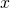in the equation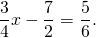For this equation, the LCD is 12, so every term in this equation will be multiplied by 12.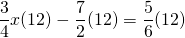Cancelling out the denominator yields: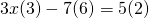Multiplying results in: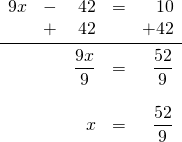Example 2.4.2

Solve forin the equation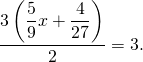First, remove the outside denominator 2 by multiplying both sides by 2: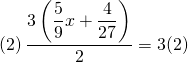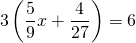Now divide both sides by 3, which leaves: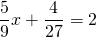To remove the 9 and 27, multiply both sides by the LCD, 27: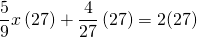This leaves: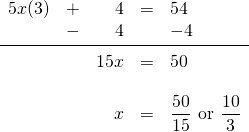# Questions

For questions 1 to 18, solve each linear equation.

1.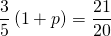2.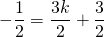3.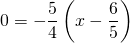4.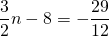5.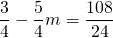6.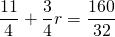7.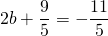8.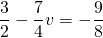9.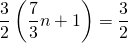10.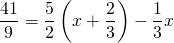11.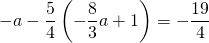12.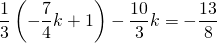13.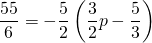14.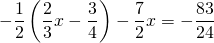15.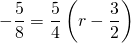16.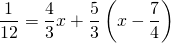17.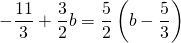18.